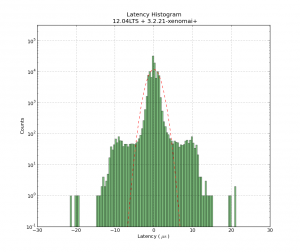# Latency HistogramThis shows a latency-histogram for a 1 ms thread running on Xenomai on my recently acquired ITX-board. Note how badly the histogram is approximated by a normal distribution (Gaussians look like parabolas with logarithmic y-scale!). See also Michael's recent RPi data and  Kent's Athlon/P4 data.

The usual latency-test numbers people report is the maximum latency, a measure of how far out to the left or right the most distant single data point lies. The histrogram can probably be used to extract many more numbers, but for real-time critical applications like cnc-machine control the maximum latency is probably an OK figure of merit.

The latency numbers are recorded with a simple HAL component:lhisto.comp

The instantaneous latency-number is then put in a FIFO by the real-time component sampler and written to a text-file using halsampler. I'm setting this up with the following HAL commands (put this in a file myfile.halrun and run with "halrun -f myfile.halrun")

loadrt sampler depth=1000 cfg=S
net latency shisto.latency sampler.0.pin.0
start
loadusr halsampler -c 0  latencysamples.txt

The numbers can now be plotted with matplotlib. I'm using the following script:

1 2 3 4 5 6 7 8 9 10 11 12 13 14 15 16 17 18 19 20 21 22 23 24 import numpy as np import matplotlib.pyplot as plt import matplotlib.mlab as mlab # load data from file x = np.loadtxt('latencysamples.txt' ) x=x/1e3 # convert to microseconds   fig = plt.figure() ax = fig.add_subplot(111) nbins = len(x)/1000 n, bins, patches = ax.hist(x, nbins, facecolor='green', alpha=0.5, log=True) bincenters = 0.5*(bins[1:]+bins[:-1]) # from matlplotlib example code mu = np.mean(x) sigma = np.std(x) area = np.trapz(n,bincenters) # scale normpdf to have the same area as the dataset y = area * mlab.normpdf( bincenters, mu, sigma) l = ax.plot(bincenters, y, 'r--', linewidth=1)# add a 'best fit' line for the normal PDF   ax.set_xlabel('Latency ( $\mathrm{ \mu s }$ ) ') ax.set_ylabel('Counts') ax.set_title('Latency Histogram\n 12.04LTS + 3.2.21-xenomai+') ax.set_ylim(1e-1, 10*max(n)) ax.grid(True) plt.show()

## 2 thoughts on “Latency Histogram”

1.Kent says:

Thanks for mentioning me.

Note that loadtxt() fails if there are any occurances of "overrun" in the captured latency data

2.anders says:

I forgot to mention the hardware for this test:
Motherboard: Intel DH61AG
CPU: Intel Core i3 2120T 2.6 GHz LGA1155

This site uses Akismet to reduce spam. Learn how your comment data is processed.